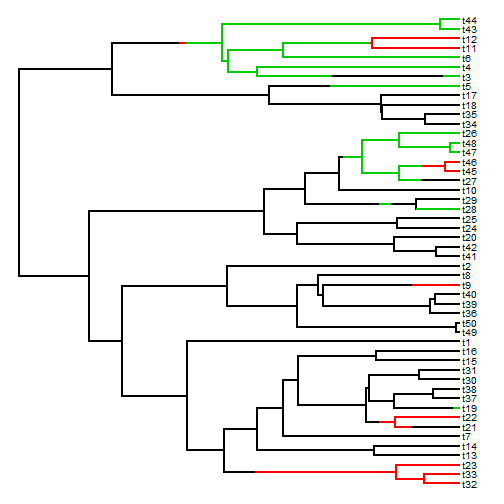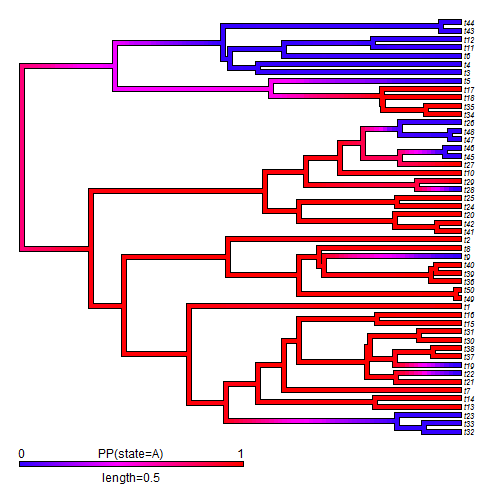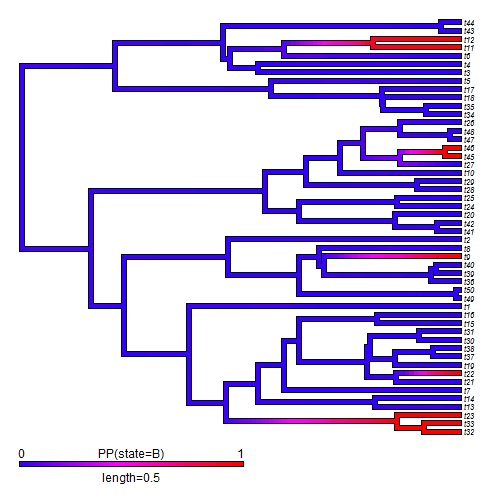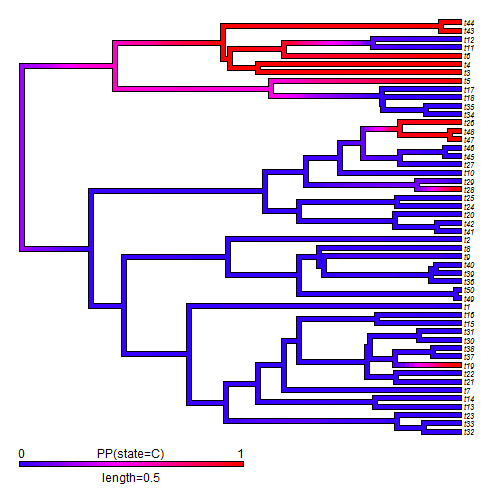## Friday, August 21, 2015

### densityMap with non-binary trait data

I (not-so) recently go the following question about the phytools function `densityMap`:

Is it possible to make same as your method 1 'Visualizing the aggregate result of stochastic mapping, in your paper (2013) : “Two new graphical methods for mapping trait evolution on phylogenies”, for a discrete trait which is not a binary trait?

Presently `densityMap`, which creates a visualization of the posterior probability density from stochastic mapping of a binary character on the tree, does not work for any more than two characters. This is mostly because it is hard to envision visualizing color in more than one dimension! (One possibly for a three-state character would be to put the probabilities on a 3D RGB scale - but this adds only one dimension to the analysis anyway.)

The only solution right now is to use `mergeMappedStates` on the `"simmap"` trees & then plot `"A"` vs. `"not A"`, `"B"` vs. `"not B"`, `"C"` vs. `"not C"`, etc.

Here is a quick demo of how to do that, which would have to be varied depending on the specific character states that were being modeled:

``````library(phytools)
packageVersion("phytools")
``````
``````##  '0.4.99'
``````
``````## simulate some data
Q<-matrix(c(-1,0.5,0.5,
0.5,-1,0.5,
0.5,0.5,-1),3,3)
rownames(Q)<-colnames(Q)<-LETTERS[1:3]
## true history
tree<-sim.history(pbtree(n=50,scale=1),Q,anc="A")
``````
``````## Done simulation(s).
``````
``````tree
``````
``````##
## Phylogenetic tree with 50 tips and 49 internal nodes.
##
## Tip labels:
##  t32, t33, t23, t13, t14, t7, ...
##
## The tree includes a mapped, 3-state discrete character with states:
##  A, B, C
##
## Rooted; includes branch lengths.
``````
``````plot(tree,fsize=0.8)
``````
``````## no colors provided. using the following legend:
##        A        B        C
##  "black"    "red" "green3"
````````````## simulated tip data
x<-getStates(tree,"tips")
``````
``````trees<-make.simmap(tree,x,nsim=100,model="ER")
``````
``````## make.simmap is sampling character histories conditioned on the transition matrix
## Q =
``````
``````##            A          B          C
## A -0.8584049  0.4292025  0.4292025
## B  0.4292025 -0.8584049  0.4292025
## C  0.4292025  0.4292025 -0.8584049
``````
``````## (estimated using likelihood);
## and (mean) root node prior probabilities
## pi =
``````
``````##         A         B         C
## 0.3333333 0.3333333 0.3333333
``````
``````## Done.
``````
``````trees
``````
``````## 100 phylogenetic trees with mapped discrete characters
``````
``````print(trees[1:10],details=TRUE)
``````
``````## 10 phylogenetic trees with mapped discrete characters
## tree 1 : 50 tips, 3 mapped states
## tree 2 : 50 tips, 3 mapped states
## tree 3 : 50 tips, 3 mapped states
## tree 4 : 50 tips, 3 mapped states
## tree 5 : 50 tips, 3 mapped states
## tree 6 : 50 tips, 3 mapped states
## tree 7 : 50 tips, 3 mapped states
## tree 8 : 50 tips, 3 mapped states
## tree 9 : 50 tips, 3 mapped states
## tree 10 : 50 tips, 3 mapped states
``````
``````## merge B & C
tBC<-lapply(trees,mergeMappedStates,c("B","C"),"B or C")
class(tBC)<-c("multiSimmap","multiPhylo")
print(tBC[1:10],details=TRUE)
``````
``````## 10 phylogenetic trees with mapped discrete characters
## tree 1 : 50 tips, 2 mapped states
## tree 2 : 50 tips, 2 mapped states
## tree 3 : 50 tips, 2 mapped states
## tree 4 : 50 tips, 2 mapped states
## tree 5 : 50 tips, 2 mapped states
## tree 6 : 50 tips, 2 mapped states
## tree 7 : 50 tips, 2 mapped states
## tree 8 : 50 tips, 2 mapped states
## tree 9 : 50 tips, 2 mapped states
## tree 10 : 50 tips, 2 mapped states
``````
``````obj<-densityMap(tBC,plot=FALSE,states=c("B or C","A"))
``````
``````## sorry - this might take a while; please be patient
``````
``````obj
``````
``````## Object of class "densityMap" containing:
##
## (1) A phylogenetic tree with 50 tips and 49 internal nodes.
##
## (2) The mapped posterior density of a discrete binary character with states (B or C, A).
``````
``````plot(obj,outline=TRUE,lwd=4,fsize=c(0.7,1))
``````Providing the argument `states=c("B or C","A")` is an important undocumented detail here, because otherwise branches of high posterior probability would be high posterior probability of `"B or C"`!

Similarly:

``````tAC<-lapply(trees,mergeMappedStates,c("A","C"),"A or C")
class(tAC)<-c("multiSimmap","multiPhylo")
obj<-densityMap(tAC,plot=FALSE,states=c("A or C","B"))
``````
``````## sorry - this might take a while; please be patient
``````
``````obj
``````
``````## Object of class "densityMap" containing:
##
## (1) A phylogenetic tree with 50 tips and 49 internal nodes.
##
## (2) The mapped posterior density of a discrete binary character with states (A or C, B).
``````
``````plot(obj,outline=TRUE,lwd=4,fsize=c(0.7,1))
````````````tAB<-lapply(trees,mergeMappedStates,c("A","B"),"A or B")
class(tAB)<-c("multiSimmap","multiPhylo")
obj<-densityMap(tAB,plot=FALSE,states=c("A or B","C"))
``````
``````## sorry - this might take a while; please be patient
``````
``````obj
``````
``````## Object of class "densityMap" containing:
##
## (1) A phylogenetic tree with 50 tips and 49 internal nodes.
##
## (2) The mapped posterior density of a discrete binary character with states (A or B, C).
``````
``````plot(obj,outline=TRUE,lwd=4,fsize=c(0.7,1))
``````Thanks for the question!

1.How to change the color of the density map?

1.Look at the function setMap.

2.Thanks. This helped too: http://blog.phytools.org/2014/12/inverting-color-map-on-object-of-class.html

2.This comment has been removed by the author.

Note: due to the very large amount of spam, all comments are now automatically submitted for moderation.